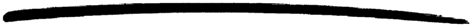# queue

queue

### MODULE SUMMARY

Abstract Data Type for FIFO Queues

### DESCRIPTION

This module implements FIFO queues in an efficient manner.

All operations has an amortised O(1) running time, except len/1, reverse/1, join/2 and split/2 that probably are O(n).

### EXPORTS

Types:

Item = term()
Q1 = Q2 = queue()

Inserts Item at the head of queue Q1. Returns the new queue Q2.

The same as last(Q) and the opposite of head(Q).

Types:

L = list()

Returns a queue containing the items in L, in the same order - the head item of the list will be the head item of the queue.

Types:

Item = term()
Q = queue()

Returns Item from the head of queue Q.

Fails with reason empty if Q is empty.

Types:

Item = term()
Q1 = Q2 = queue()

Inserts Item at the tail of queue Q1. Returns a new queue Q2. This is the same as snoc(Q1, Item).

Types:

Item = term()
Q1 = Q2 = queue()

Inserts Item at the head of queue Q1. Returns a new queue Q2. This is the same as cons(Item, Q1).

Types:

Item = term()
Q1 = Q2 = queue()

Returns a queue Q2 that is the result of removing the last item from Q1. This is the opposite of tail(Q1).

Fails with reason empty if Q1 is empty.

Types:

Q = queue()

Tests if Q is empty and returns true if so and false otherwise.

Types:

Q1 = Q2 = Q3 = queue()

Returns a queue Q3 that is the result of joining Q1 and Q2 with Q1 before (at the head) Q2.

The same as init(Q1) and the opposite of tail(Q1).

Types:

Item = term()
Q = queue()

Returns the last item of queue Q. This is the opposite of head(Q).

Fails with reason empty if Q is empty.

Types:

Q = queue()
N = integer()

Calculates and returns the length of queue Q.

Types:

Q = queue()

Returns an empty queue.

Types:

Result = {{value, Item}, Q2} | {empty, Q1}
Q1 = Q2 = queue()

Removes the head item from the queue Q1. Returns the tuple {{value, Item}, Q2}, where Item is the item removed and Q2 is the new queue. If Q1 is empty, the tuple {empty, Q1} is returned.

Types:

Result = {{value, Item}, Q2} | {empty, Q1}
Q1 = Q2 = queue()

Removes the last item from the queue Q1. Returns the tuple {{value, Item}, Q2}, where Item is the item removed and Q2 is the new queue. If Q1 is empty, the tuple {empty, Q1} is returned.

Types:

Q1 = Q2 = queue()

Returns a queue Q2 that contains the items of Q1 in the reverse order.

Types:

Item = term()
Q1 = Q2 = queue()

Inserts Item as the last item of queue Q1. Returns the new queue Q2. This is the opposite of cons(Item, Q1).

Types:

N = integer()
Q1 = Q2 = Q3 = queue()

Splits Q1 into a queue Q2 of length N with items from the head end, and a queue Q3 with the rest of the items.

Types:

Item = term()
Q1 = Q2 = queue()

Returns a queue Q2 that is the result of removing the head item from Q1.

Fails with reason empty if Q1 is empty.

Types:

Q = queue()

Returns a list of the items in the queue, with the head item of the queue as the head of the list.

stdlib 1.15
Copyright © 1991-2007 Ericsson AB